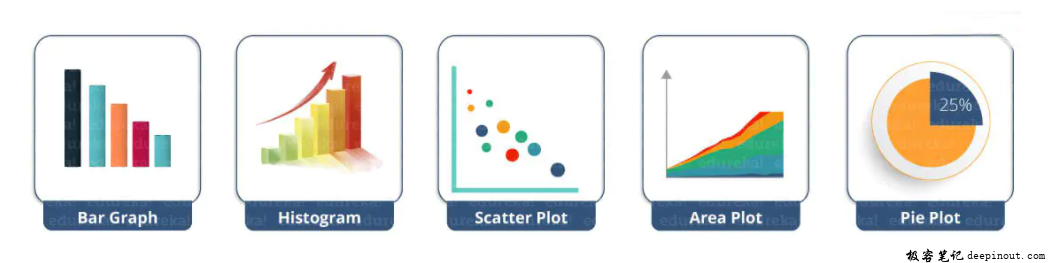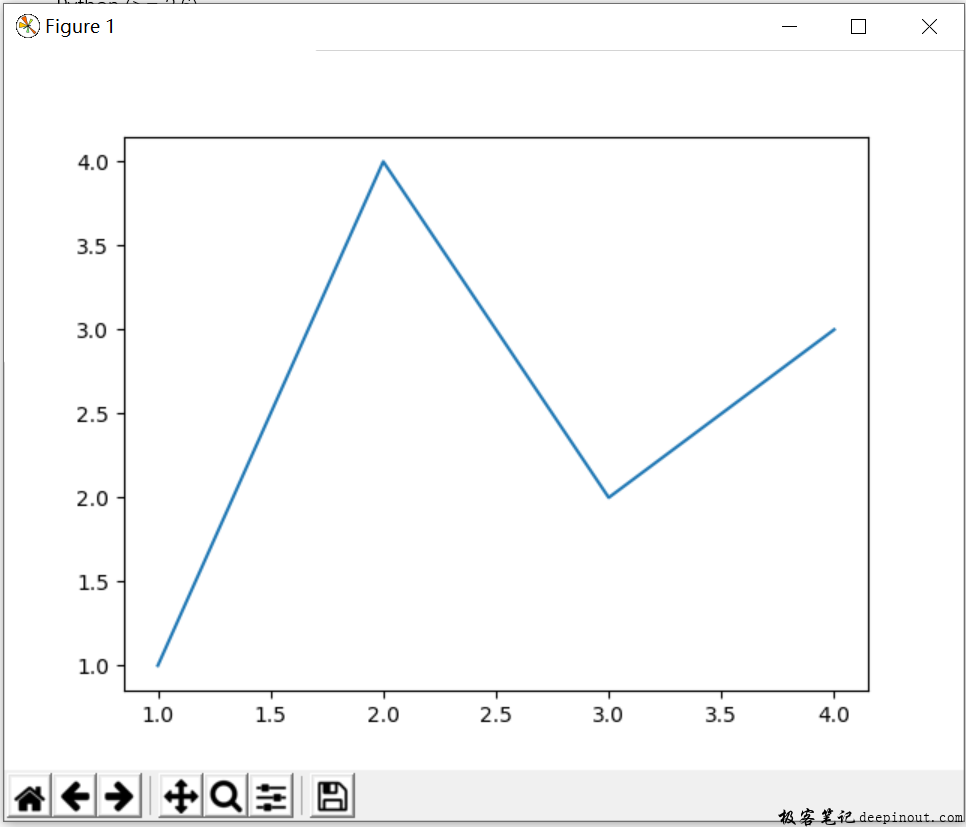# Matplotlib 入门

matplotlib库是Python中绘制二维、三维图表的数据可视化工具。它的主要特点如下。

• 使用简单绘图语句实现复杂绘图效果；

• 以交互式操作实现渐趋精细的图形效果；

• 使用嵌入式的LaTeX输出具有印刷级别的图表、科学表达式和符号文本；

• 对图表的组成元素实现精细化控制。

Matplotlib 是一个 Python 的 2D绘图库，它以各种硬拷贝格式和跨平台的交互式环境生成出版质量级别的图形。通过 Matplotlib，开发者可以仅需要几行代码，便可以生成绘图，直方图，功率谱，条形图，错误图，散点图等。

## 为什么选择 Matplotlib

### Matplotlib 能帮你

Matplotlib是Python中用的最多的2D图形绘图库，学好Matplotlib的用法可以帮助我们在统计分析中更灵活的展示各种数据的状态。这是数据可视化必学的库，好好学吧。``````python -m pip install -U pip
python -m pip install -U matplotlib
``````

Matplotlib 依赖下面的库:

• Python (>= 3.6)
• NumPy (>= 1.15)
• setuptools
• cycler (>= 0.10.0)
• dateutil (>= 2.1)
• kiwisolver (>= 1.0.0)
• Pillow (>= 6.2)
• pyparsing (>=2.0.3)

## 第一个Matplotlib 测试例子

``````import matplotlib.pyplot as plt
import numpy as np

fig, ax = plt.subplots()  # 创建一张白纸figure，包含一个坐标系.

ax.plot([1, 2, 3, 4], [1, 4, 2, 3])  # 在坐标系画图.

plt.show()
``````• 微信订阅
• 回顶